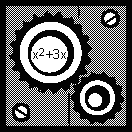# 3.7 Function concepts -- function notation

 Page 1 / 1
This module describes notation for functions.

## Function notation

Functions are represented in math by parentheses. When you write $f\left(x\right)$ you indicate that the variable $f$ is a function of—or depends on—the variable $x$ .

For instance, suppose $f\left(x\right)={x}^{2}+3x$ . This means that f is a function that takes whatever you give it, and squares it, and multiplies it by 3, and adds those two quantities.

 $\begin{array}{c}7\to \\ \text{10}\to \\ x\to \\ y\to \\ \text{a dog}\to \end{array}$$\begin{array}{c}\to f\left(7\right)={7}^{2}+3\left(7\right)=\text{70}\\ \to f\left(\text{10}\right)={\text{10}}^{2}+3\left(\text{10}\right)=\text{130}\\ \to f\left(x\right)={x}^{2}+3x\\ \to f\left(y\right)={y}^{2}+3y\\ \begin{array}{}\to f\left(\text{dog}\right)={\left(\text{dog}\right)}^{2}+3\left(\text{dog}\right)\\ \left(\text{*not in the domain}\right)\end{array}\end{array}$

The notation $f\left(7\right)$ means “plug the number 7 into the function $f$ .” It does not indicate that you are multiplying $f$ times 7. To evaluate $f\left(7\right)$ you take the function $f\left(x\right)$ and replace all occurrences of the variable x with the number 7. If this function is given a 7 it will come out with a 70.

If we write $f\left(y\right)={y}^{2}+3y$ we have not specified a different function . Remember, the function is not the variables or the numbers, it is the process. $f\left(y\right)={y}^{2}+3y$ also means “whatever number comes in, square it, multiply it by 3, and add those two quantities.” So it is a different way of writing the same function.

Just as many students expect all variables to be named $x$ , many students—and an unfortunate number of parents—expect all functions to be named $f$ . The correct rule is that—whenever possible— functions, like variables, should be named descriptively . For instance, if Alice makes \$100/day, we might write:

• Let m equal the amount of money Alice has made (measured in dollars)
• Let t equal the amount of time Alice has worked (measured in days)
• Then, $m\left(t\right)=\text{100}t$

This last equation should be read “ $m$ is a function of $t$ (or $m$ depends on $t$ ). Given any value of the variable $t$ , you can multiply it by 100 to find the corresponding value of the variable $m$ .”

Of course, this is a very simple function! While simple examples are helpful to illustrate the concept, it is important to realize that very complicated functions are also used to model real world relationships. For instance, in Einstein’s Special Theory of Relativity, if an object is going very fast, its mass is multiplied by $\frac{1}{\sqrt{1-\frac{{v}^{2}}{9\cdot {\text{10}}^{\text{16}}}}}$ . While this can look extremely intimidating, it is just another function. The speed $v$ is the independent variable, and the mass $m$ is dependent. Given any speed $v$ you can determine how much the mass $m$ is multiplied by.

a diagram of an adult mosquito
what are white blood cells
white blood cell is part of the immune system. that help fight the infection.
MG
Mlungisi
Cells with a similar function, form a tissue. For example the nervous tissue is composed by cells:neurons and glia cells. Muscle tissue, is composed by different cells.
Anastasiya
I need further explanation coz celewi anything guys,,,
hey guys
Isala
on what?
Anastasiya
is air homogenous or hetrogenous
homogenous
Kevin
why saying homogenous?
Isala
explain if oxygen is necessary for photosynthesis
explain if oxygen is necessary for photosynthesis
Yes, the plant does need oxygen. The plant uses oxygen, water, light, and produced food. The plant use process called photosynthesis.
MG
By using the energy of sunlight, plants convert carbon dioxide and water into carbohydrates and oxygen by photosynthesis. This happens during the day and sunlight is needed.
NOBLE
no. it s a product of the process
Anastasiya
yet still is it needed?
NOBLE
no. The reaction is: 6CO2+6H20+ solar energy =C6H12O6(glucose)+602. The plant requires Carbon dioxyde, light, and water Only, and produces glucose and oxygen( which is a waste).
Anastasiya
what was the question
joining
Godfrey
the specific one
NOBLE
the study of non and living organism is called.
Godfrey
Is call biology
Alohan
yeah
NOBLE
yes
Usher
what Is ecology
what is a cell
A cell is a basic structure and functional unit of life
Ndongya
what is biolgy
is the study of living and non living organisms
Ahmed
may u draw the female organ
i dont understand
Asal
:/
Asal
me too
DAVID
anabolism and catabolism
Anabolism refers to the process in methabolism in which complex molecules are formed "built" and requires energy to happen. Catabolism is the opposite process: complex molecules are deconstructed releasing energy, such as during glicolysis.
Anastasiya
Explain briefly independent assortment gene .
hi
Amargo
hi I'm Anatalia
Joy
what do you mean by pituitary gland
Digambar
draw and lable the cell
why homones are destroyed soon after completing their role
Got questions? Join the online conversation and get instant answers!By David CoreyBy OpenStaxBy OpenStaxBy Ryan LoweBy John GabrieliBy Yacoub JayoghliBy Robert MurphyBy Abishek DevarajBy Anh DaoBy OpenStax